# 李宏毅深度学习_Backpropagation

## Background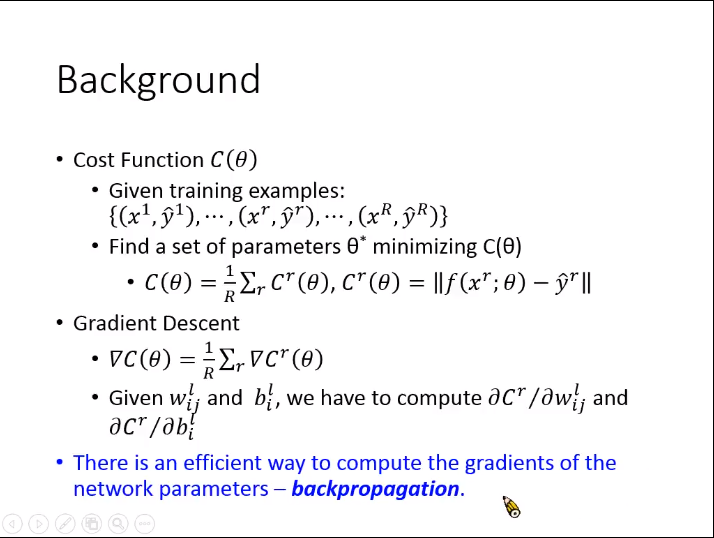## 链式法则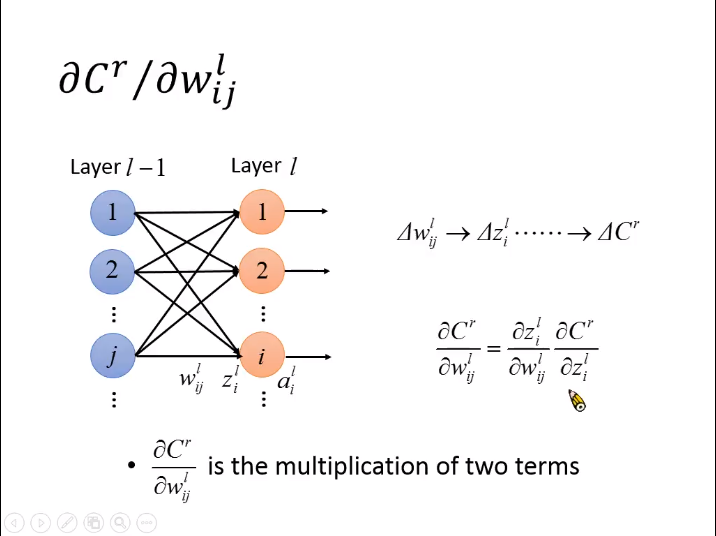## 计算链式法则中拆解的两部分

1. 计算$\mathrm{\partial }{z}_{i}^{l}/\mathrm{\partial }{w}_{ij}^{l}$$\partial z^l_i/ \partial w^l_{ij}$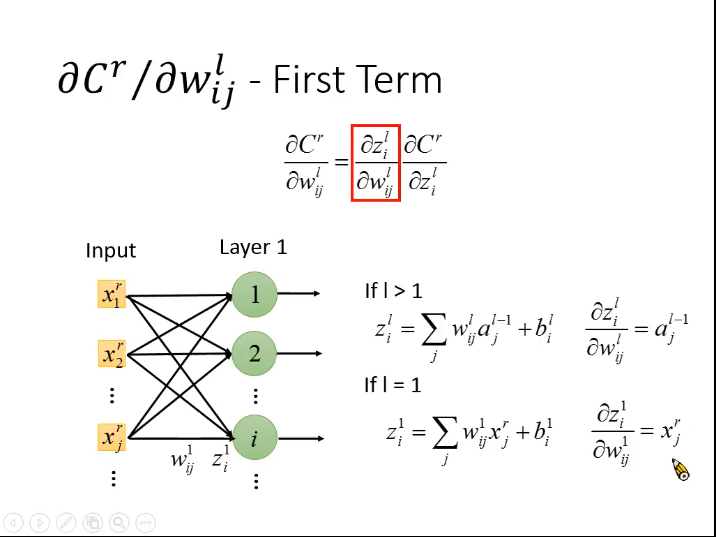• $l>1$$l>1$。即当权值不是第一层时，导数为${a}_{j}^{l-1}$$a^{l-1}_j$
• $l=1$$l=1$。即权值为第一层时，导数为${x}_{j}^{r}$$x^r_j$

2. 计算$\mathrm{\partial }{C}^{r}/\mathrm{\partial }{z}_{i}^{l}$$\partial C^r/ \partial z^l_{i}$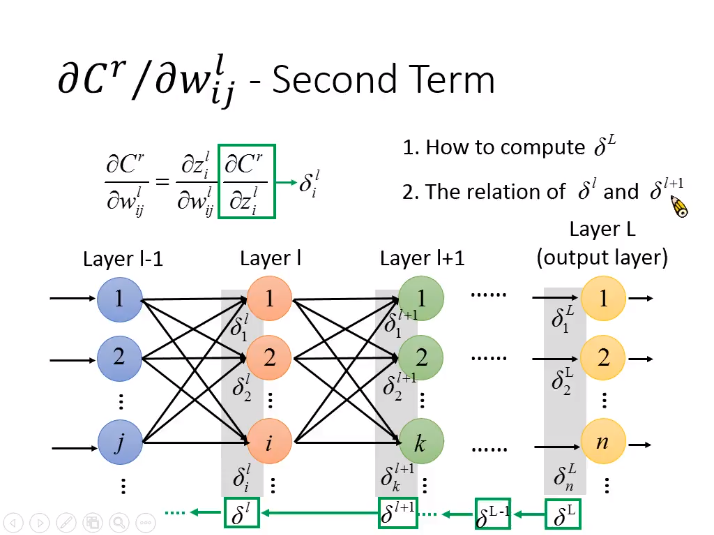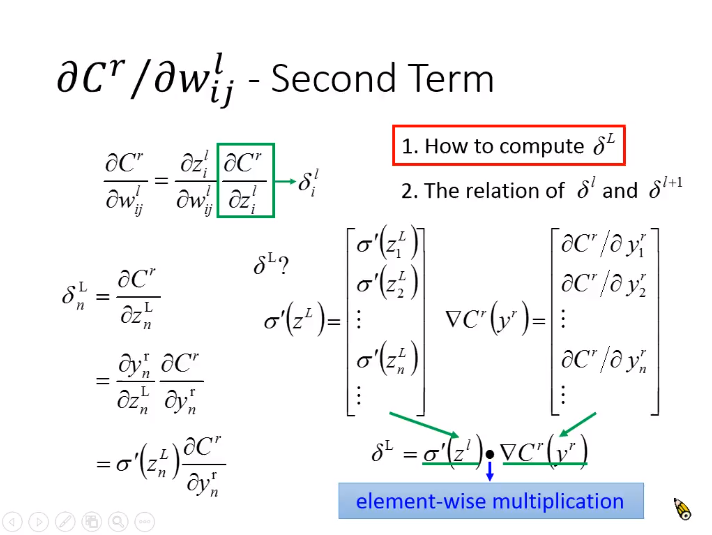${\delta }_{n}^{L}={\sigma }^{\prime }\left({z}_{n}^{L}\right)\frac{\mathrm{\partial }{C}^{r}}{\mathrm{\partial }{y}_{n}^{r}}$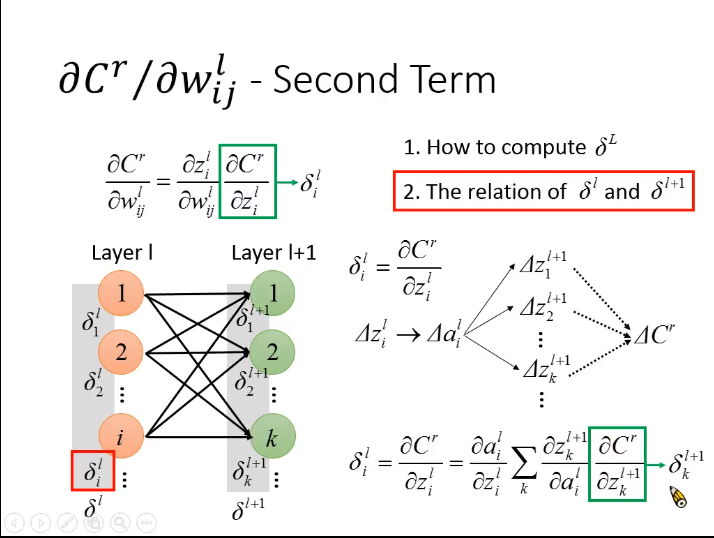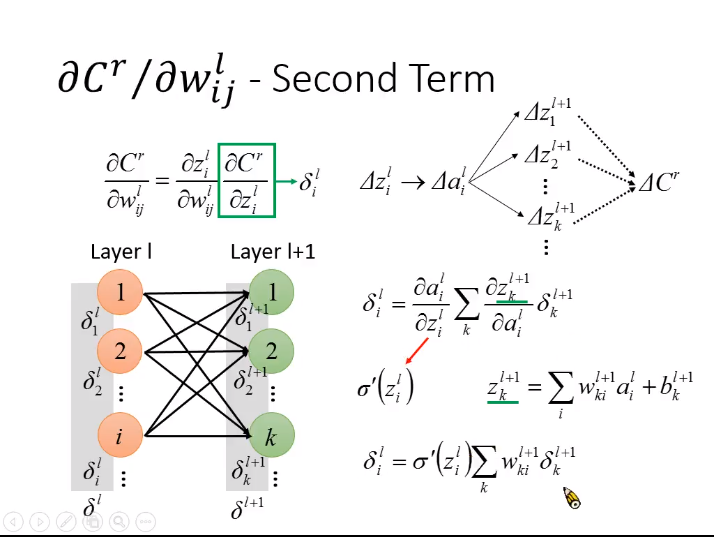${z}_{i}^{l}$$z^l_{i}$的变化$\mathrm{\Delta }{z}_{i}^{l}$$\Delta z^l_{i}$会对${a}_{i}^{l}$$a^l_{i}$造成影响进而影响到下一层的${z}^{l+1}$$z^{l+1}$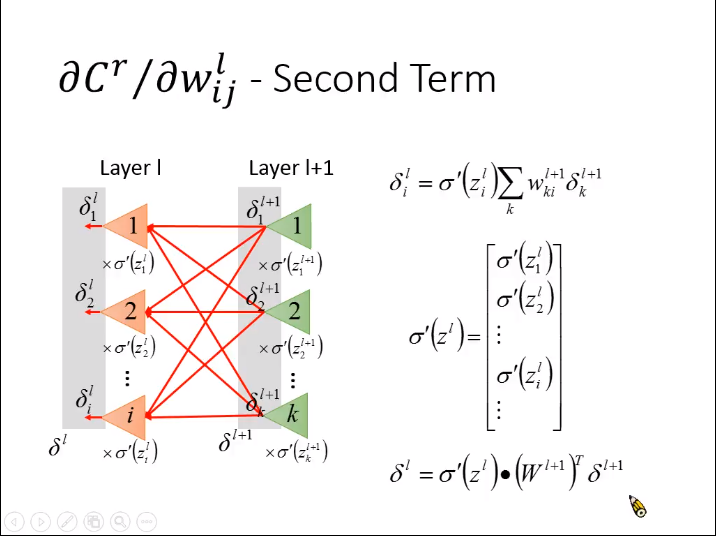${\delta }^{l}={\sigma }^{\prime }\left({z}^{l}\right)\cdot \left({W}^{l+1}{\right)}^{T}{\delta }^{l+1}。$

## 总结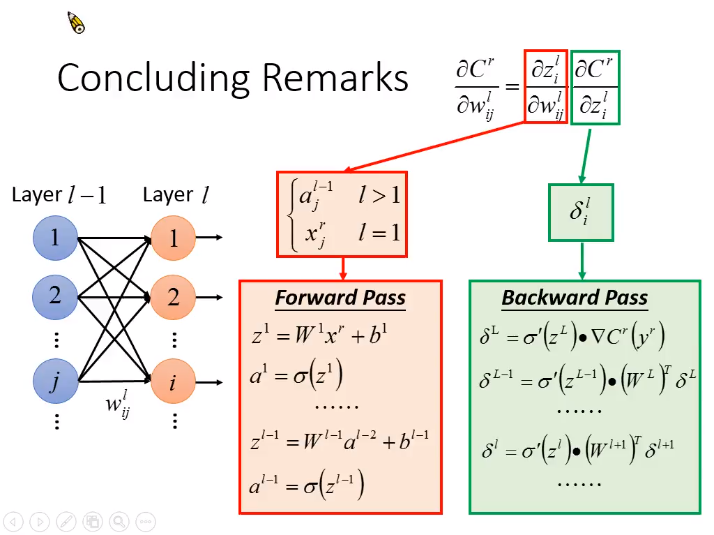©️2019 CSDN 皮肤主题: 大白 设计师: CSDN官方博客